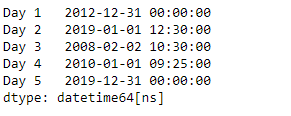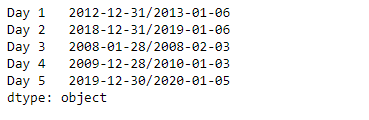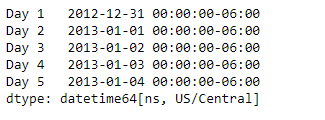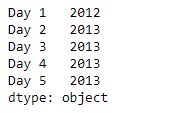# Python | Pandas Series.dt.to_period

`Series.dt` can be used to access the values of the series as datetimelike and return several properties. Pandas` Series.dt.to_period()` function cast the underlying data of the given Series object to PeriodArray/Index at a particular frequency.

Syntax: Series.dt.to_period(*args, **kwargs)

Parameter :

freq : string or Offset, optional

Returns : PeriodArray/Index

Example #1: Use `Series.dt.to_period()` function to cast the underlying data of the given series object to Index at Weekly frequency.

 `# importing pandas as pd ` `import` `pandas as pd ` ` `  `# Creating the Series ` `sr ``=` `pd.Series([``'2012-12-31'``, ``'2019-1-1 12:30'``, ``'2008-02-2 10:30'``, ` `               ``'2010-1-1 09:25'``, ``'2019-12-31 00:00'``]) ` ` `  `# Creating the index ` `idx ``=` `[``'Day 1'``, ``'Day 2'``, ``'Day 3'``, ``'Day 4'``, ``'Day 5'``] ` ` `  `# set the index ` `sr.index ``=` `idx ` ` `  `# Convert the underlying data to datetime  ` `sr ``=` `pd.to_datetime(sr) ` ` `  `# Print the series ` `print``(sr) `

Output :Now we will use `Series.dt.to_period()` function to cast the underlying data of the given series object to Index at Weekly frequency.

 `# cast to targert frequency ` `result ``=` `sr.dt.to_period(freq ``=` `'W'``)  ` ` `  `# print the result ` `print``(result) `

Output :As we can see in the output, the `Series.dt.to_period()` function has successfully cast the data to the target frequency.

Example #2 : Use `Series.dt.to_period()` function to cast the underlying data of the given series object to Index at two year frequency.

 `# importing pandas as pd ` `import` `pandas as pd ` ` `  `# Creating the Series ` `sr ``=` `pd.Series(pd.date_range(``'2012-12-31 00:00'``, periods ``=` `5``, freq ``=` `'D'``, ` `                            ``tz ``=` `'US / Central'``)) ` ` `  `# Creating the index ` `idx ``=` `[``'Day 1'``, ``'Day 2'``, ``'Day 3'``, ``'Day 4'``, ``'Day 5'``] ` ` `  `# set the index ` `sr.index ``=` `idx ` ` `  `# Print the series ` `print``(sr) `

Output :Now we will use `Series.dt.to_period()` function to cast the underlying data of the given series object to Index at two year frequency.

 `# cast to targert frequency ` `result ``=` `sr.dt.to_period(freq ``=` `'2Y'``)  ` ` `  `# print the result ` `print``(result) `

Output :As we can see in the output, the `Series.dt.to_period()` function has successfully cast the data to the target frequency.

My Personal Notes arrow_drop_upCheck out this Author's contributed articles.

If you like GeeksforGeeks and would like to contribute, you can also write an article using contribute.geeksforgeeks.org or mail your article to contribute@geeksforgeeks.org. See your article appearing on the GeeksforGeeks main page and help other Geeks.

Please Improve this article if you find anything incorrect by clicking on the "Improve Article" button below.

Article Tags :

Be the First to upvote.

Please write to us at contribute@geeksforgeeks.org to report any issue with the above content.